Open in App
Not now

# How to utilise timeseries in pandas?

• Last Updated : 28 Feb, 2022

An ordered stream of values for a variable at evenly spaced time periods is known as a time series. Timeseries are useful in identifying the underlying factors and structures that resulted in the observed data and After you’ve fitted a model, one can move on to forecasting, monitoring. some applications of time series are Analysis of the Stock Market, Estimated Yields, studies of the spread of diseases like covid19 etc. We can use time series to a particular data based on certain conditions. In this article let’s demonstrate how to use time-series data.

## Utilize timeseries in Pandas

All the examples are made on covid_19 data. After importing the CSV file ‘ObservationDate’ and ‘Last Update’ dates are converted to datetime using pd.to_datetime() method.

## Python3

 `# import packages ` `import` `pandas as pd ` ` `  `# read csv file ` `df ``=` `pd.read_csv(``'covid_19.csv'``, encoding``=``'UTF-8'``) ` ` `  `df[``'ObservationDate'``] ``=` `pd.to_datetime(df[``'ObservationDate'``]) ` `df[``'Last Update'``] ``=` `pd.to_datetime(df[``'Last Update'``]) ` `print``(df) `

Output: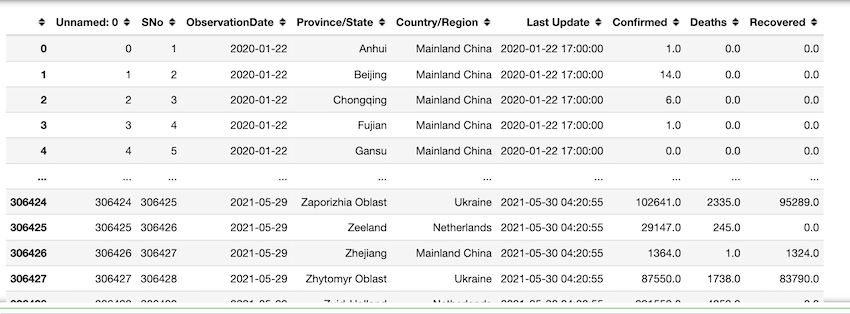Extract all observations before 2021. 192466 rows are retrieved.

## Python3

 `df[df[``'ObservationDate'``]<``=``'2021'``]`

Output: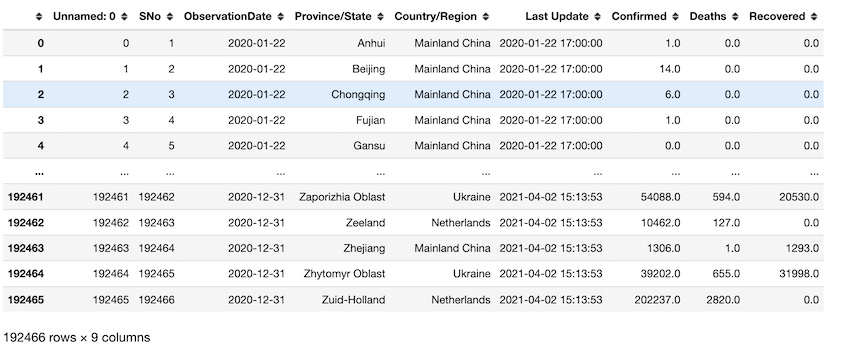Retrieving observations of a particular day. in this example, we set the day to be ‘2020-06’.

## Python3

 `df[df[``'ObservationDate'``] ``=``=` `'2020-06'``] `

Output: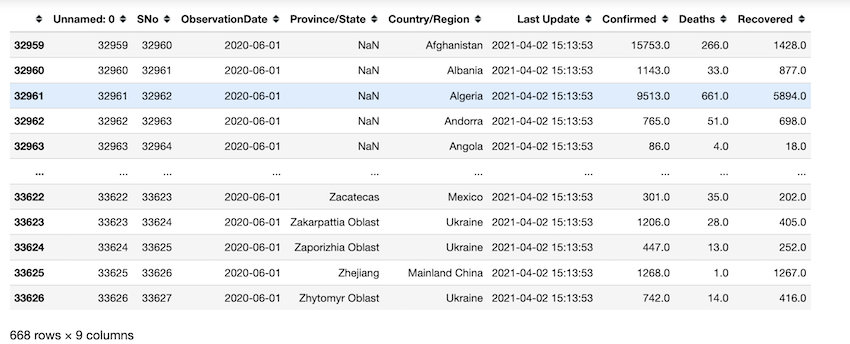Retrieving the day where maximum deaths are the highest. on 2021-05-29 maximum deaths are recorded from UK as per our data.

## Python3

 `df[df[``'Deaths'``] ``=``=` `max``(df[``'Deaths'``])] `

Output: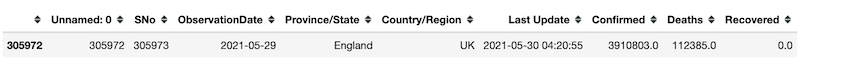Output

Sum of all the deaths on ‘2021-05-20’.

## Python3

 `sum``(df[df[``'ObservationDate'``] ``=``=` `'2021-05-20'``][``'Deaths'``]) `

Output:

`3430539.0`

Instead of working on the hard way to retrieve data, we can set time series columns to datetime and set them as the index of the dataframe to easily retrieve the information we need. ObservationDate is set as the index of the dataframe in this example. by using df.loc() we can index and access required information by dates directly. df.loc[‘2020-01’] retrieves all the data of that date. The output shows that there are 513 observations.

## Python3

 `# import packages ` `import` `pandas as pd ` ` `  `# read csv file ` `df ``=` `pd.read_csv(``'covid_19.csv'``) ` `df[``'ObservationDate'``] ``=` `pd.to_datetime(df[``'ObservationDate'``]) ` `df[``'Last Update'``] ``=` `pd.to_datetime(df[``'Last Update'``]) ` `df ``=` `df.set_index(``'ObservationDate'``) ` `print``(df.loc[``'2020-01'``]) `

Output: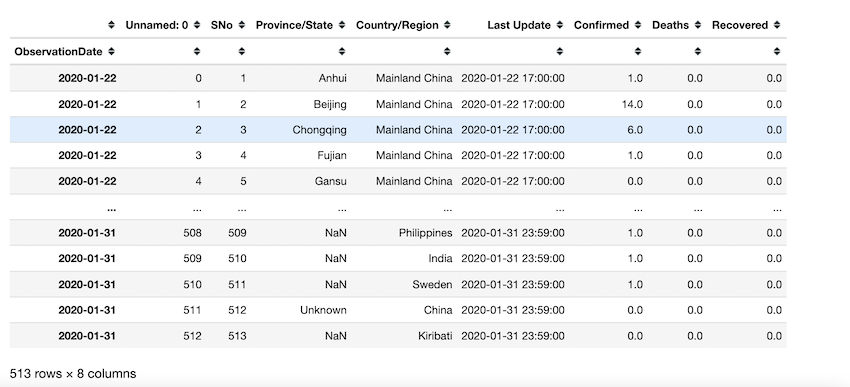Observations taken from may 20th to may 21st of 2021 are retrieved using indexing.

## Python3

 `# import packages ` `import` `pandas as pd ` ` `  `# read csv file ` `df ``=` `pd.read_csv(``'covid_19.csv'``) ` `df[``'ObservationDate'``] ``=` `pd.to_datetime(df[``'ObservationDate'``]) ` `df[``'Last Update'``] ``=` `pd.to_datetime(df[``'Last Update'``]) ` `df ``=` `df.set_index(``'ObservationDate'``) ` ` `  `# observations taken from may 20th to may 21st of 2021 ` `df.loc[``'2021-05-20'``:``'2021-05-21'``] `

Output: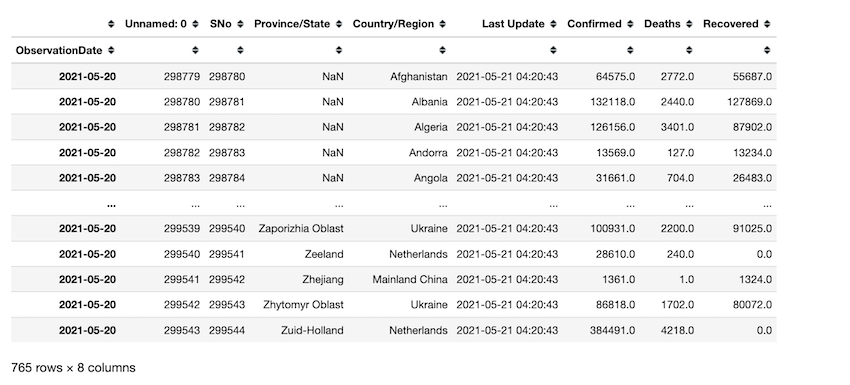In this example, df.groupby() is used to group all the observations based on the date they got updated and count them. for example, the first row says there are 40 observations on ‘2020-01-22’.

## Python3

 `# import packages ` `import` `pandas as pd ` ` `  `# read csv file ` `df ``=` `pd.read_csv(``'covid_19.csv'``) ` `df[``'ObservationDate'``] ``=` `pd.to_datetime(df[``'ObservationDate'``]) ` `df[``'Last Update'``] ``=` `pd.to_datetime(df[``'Last Update'``]) ` `df ``=` `df.set_index(``'ObservationDate'``) ` `print``(df.groupby(level``=``0``).count()) `

Output: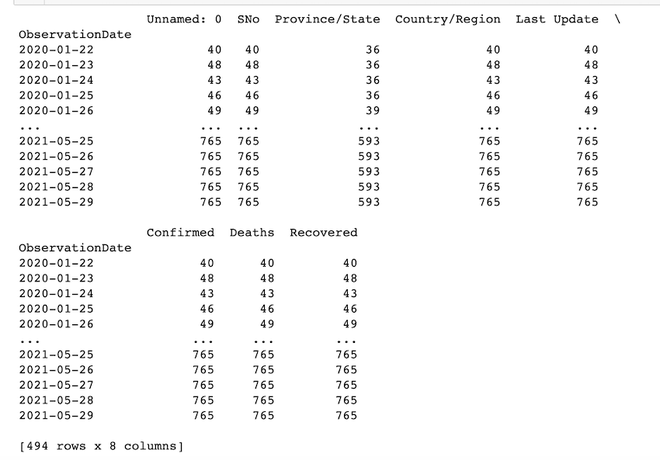After setting the index of the dataframe to time-series, we use df.plot.line() method to visualize all the information through a single line plot. Time series data helps us make good conclusions.

## Python3

 `# import packages and libraries ` `import` `pandas as pd ` `from` `matplotlib ``import` `pyplot as plt ` `import` `numpy as np ` ` `  `# reading the dataset ` `df ``=` `pd.read_csv(``'covid_19_data.csv'``, encoding``=``'UTF-8'``) ` ` `  `# convert Last update column to datetime ` `df[``'Last Update'``] ``=` `pd.to_datetime(df[``'Last Update'``]) ` ` `  `# setting index ` `df.set_index(``'Last Update'``, inplace``=``True``) ` ` `  `# plotting figure ` `df.plot.line() `

Output: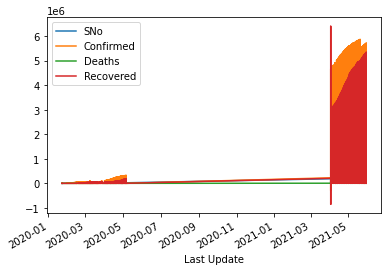My Personal Notes arrow_drop_up
Related Articles